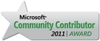We are excited to announce that the ASP.NET Forums are moving to the new Microsoft Q&A experience. Learn more >

## 3 replies

Last post Oct 13, 2016 11:16 AM by eralper

• ### district name

```ALTER procedure [dbo].[spGetpraptra11rptByDate]
(
@fromdate Date,
@todate Date

)
as
Begin
select
sum(p1.cell_02) as cell_02,
sum(p1.cell_03) as cell_03,
sum(p1.cell_04) as cell_04,
sum(p1.cell_05) as cell_05,
sum(p1.cell_06) as cell_06,
sum(p1.cell_07) as cell_07,
sum(p1.cell_08) as cell_08,
sum(p1.cell_09) as cell_09,
sum(p1.cell_10) as cell_10,
sum(p1.cell_11) as cell_11,
sum(p1.cell_12) as cell_12,
sum(p1.cell_13) as cell_13,
sum(p1.cell_14) as cell_14,
sum(p1.cell_15) as cell_15,
sum(p1.cell_16) as cell_16,
sum(p1.cell_17) as cell_17,
sum(p1.cell_18) as cell_18,
sum(p1.cell_19) as cell_19,
sum(p1.cell_20) as cell_20,
sum(p1.cell_21) as cell_21,
sum(p1.cell_22) as cell_22,
sum(p1.cell_23) as cell_23,
sum(p1.cell_24) as cell_24,
sum(p1.cell_25) as cell_25,
sum(p1.cell_26) as cell_26,
sum(p1.cell_27) as cell_27,
sum(p1.cell_28) as cell_28,
sum(p1.cell_29) as cell_29,
sum(p1.cell_30) as cell_30,
sum(p1.cell_31) as cell_31,
sum(p1.cell_32) as cell_32,
sum(p1.cell_33) as cell_33,
sum(p1.cell_34) as cell_34,

c.CircleHName   from praptra11 p1, tblCircle c where  p1.CircleId=c.CircleId and
(p1.r_date between @fromdate and @todate) group by c.CircleHName;
select p1.*, convert(varchar, r_date, 103) as EDate,c.CircleHName from praptra11 p1, tblCircle c where  p1.CircleId=c.CircleId
and (p1.r_date between @fromdate and @todate) order by p1.CircleId

End```

above is my procedure.

i want to get district name in above procedure through [tblDistrict] table. how to modify above procedure.

SELECT [DistrictId]
,[DistrictName]
,[DistrictHName]
,[Status]
,[CDate]
,[CBy]
FROM [tblDistrict]

• ### Re: district name

Hello mike,

How can we relate the district table with the table and columns used in the SELECT statements of the procedure?

Is there a districtid column in tblCircle table?

If so then we will join these related tables using the foreign key columns

T-SQL programming SQL Server Tutorials
SQL Server 2017• ### Re: district name

Is there a districtid column in tblCircle table?

yes i have districtid column in tblCircle table

• ### Re: district name

```select
.......
sum(p1.cell_33) as cell_33,
sum(p1.cell_34) as cell_34,
c.CircleHName,
d.DistrictName
from	praptra11 p1,
tblCircle c,
tblDistrict d
where  p1.CircleId=c.CircleId and
c.DistrictId=d.DistrictId and
(p1.r_date between @fromdate and @todate)
group by c.CircleHName, d.DistrictName;

select
p1.*, convert(varchar, r_date, 103) as EDate,c.CircleHName, d.DistrictName
from praptra11 p1, tblCircle c, tblDistrict d
where  p1.CircleId=c.CircleId
and c.DistrictId=d.DistrictId
and (p1.r_date between @fromdate and @todate)
order by p1.CircleId
```

I hope it helps

T-SQL programming SQL Server Tutorials
SQL Server 2017